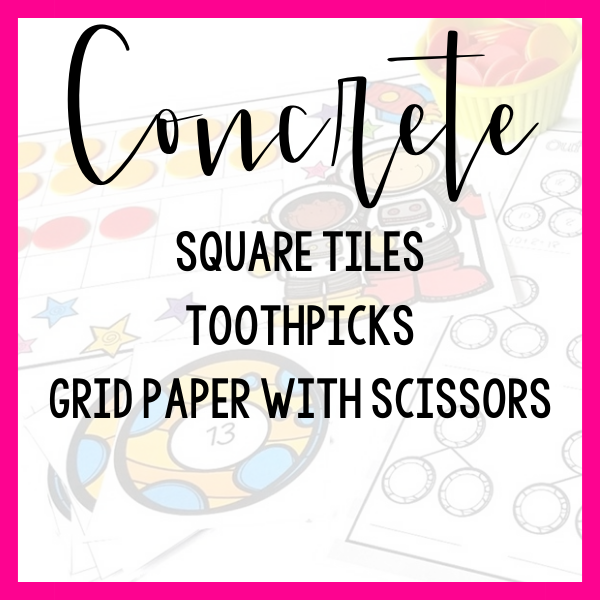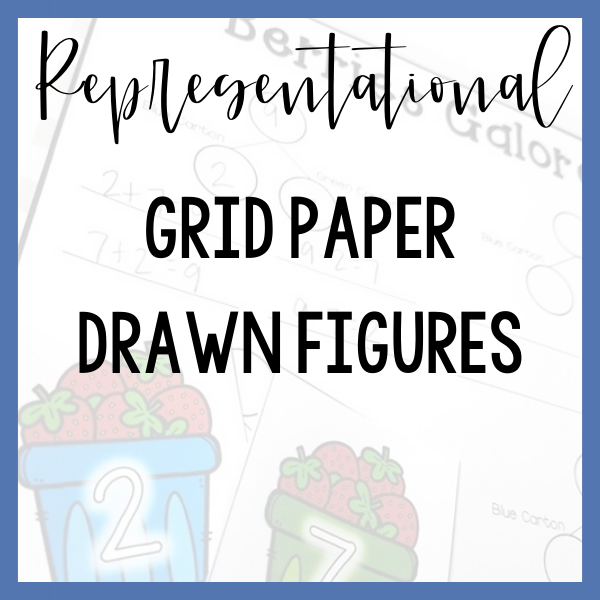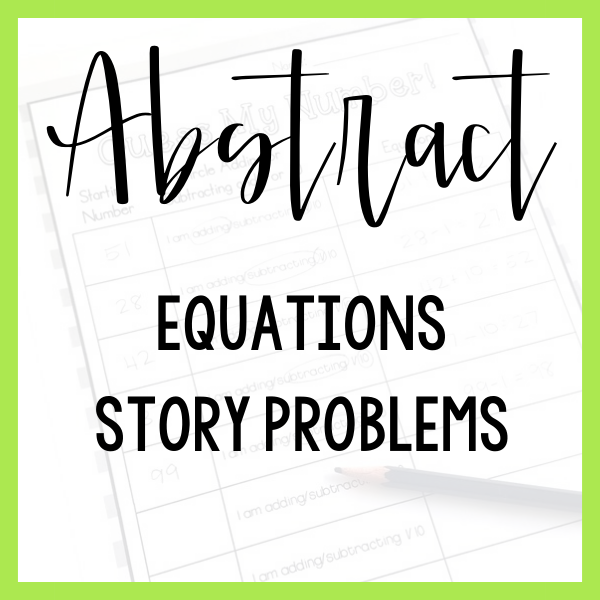# Area and Perimeter

Third grade students are asked to explore the idea of area from three main lenses.

First, that area is the number of unit squares that can cover a space- students explore this dimension of area by tiling to find the area of a rectangle.

Next, students explore the connection between arrays and area of a rectangle.

Finally, students explore the idea that area is additive and that multiples rectangles can be put together (without overlapping) to create a larger area. Conversely, a shape can be decomposed into non-overlapping rectangles and by finding the area of each of these rectangles you can find the area of the whole.

3rd grade students also explore the idea of perimeter, solve word problems involving perimeter and explore the relationship between area and perimeter.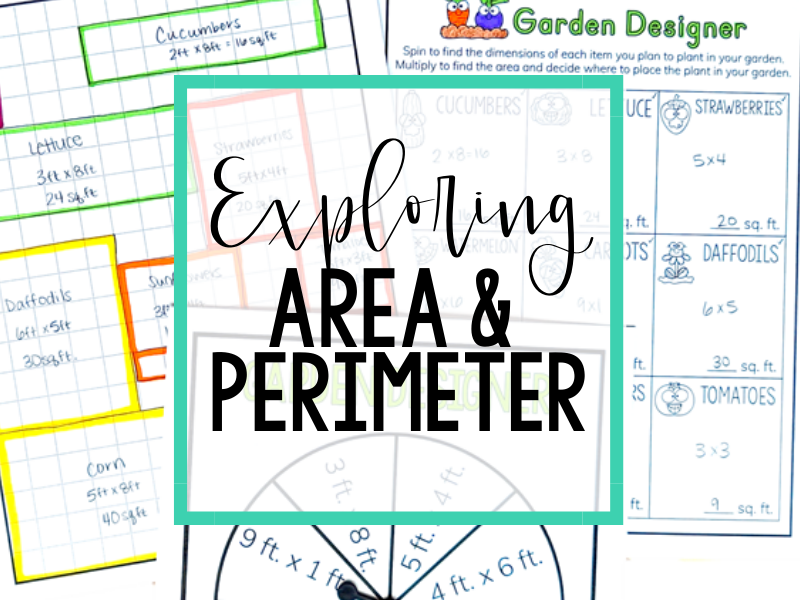The 5 examples listed are certainly not an exhaustive list of tools and lessons that could be used to teach this skill.

They are rather a sample progression from hands-on to abstract thinking!

Which step represents your students’ current level of understanding?

Provide your students with a rectangle with whole number length sides (ex: A 3″x5″ rectangle). Tell your students simply that you want them to figure out how many squares it would take to cover the rectangle entirely. Allow your students to make predictions of how many squares it will take to cover the rectangle.

As your students are working together to cover the rectangle with squares, periodically pause to notice and prompt, for example:

• I notice that your squares are lined up without any space between them, that’s going to give you a very accurate measurement, great job!
• Or, conversely: I notice that there is space between your squares, let’s move them close together so that they are touching so that we can measure the whole area!
• Let’s pause and think about our predictions. Do you still predict it will take the same number of squares to cover the rectangle or would you like to adjust your prediction?
• Do these rows of squares remind you of anything? Yes, they look like an array to me too!

After your students have covered the rectangle, tell them that you want to introduce a new vocabulary word, Area!

Repeat the activity with a variety of rectangles asking your students to use the square tiles to find the area of the rectangles provided.

Start by presenting your students with a math story: I am building a table that will be 6 ft long and 3 ft wide, I’m wondering what the area of that table will be so I can see if it will fit into my dining room. Let’s draw a picture and see if we can think this through!

Work together with your students to draw a picture or model that matches the story.

Before moving on, ask your students if they have any idea how you might be able to find the area of the table – your students may surprise you with their connections to the previous lesson!

Remind your students that yesterday you used square tiles to find the area. Work as a group to build a model that matches the story using square tiles.

Next, ask your students if they notice any similarities between the drawing you created as a group and the model you built with square tiles. Ask your students to now find the area of the rectangle  using the model with square tiles to confirm their calculation.

At this point, be explicit, ask your students “What multiplication equation helps us find the area of a rectangle with a length of 6 ft and a width of 3 ft?” Record 6 x 3 = 18 on a white board. Pose the question, “I wonder, do you think multiplying the length and width of a rectangle will always tell us the area of the rectangle?” and allow your students to discuss.

The purpose of this lesson is for your students to understand that a rectilinear figure can be decomposed so that you can find the area of each rectangle and then added back together to find the total area of the figure.

Presenting your students with a rectilinear figure (have 3 copies of this figure available) and a pair of scissors, ask your students if there is any you might be able to break figure into two rectangles so that it is easier to calculate.

Note, the figure can be divided in multiple ways! Your students may suggest dividing the figure horizontally or vertically- either is fine!

After cutting the figure, pass each section of the figure to a small group of students and ask them to find the area of their rectangle labeling the area on their rectangle.

Take the rectangles back and glue them back together onto a piece of construction paper and askyour students to add the two areas together to find the total area of the figure.

Next, repeat the same activity with the same figure dividing the figure into rectangles a new way. Notice that it doesn’t matter how you decompose the rectangle, as long as you are finding the area of all parts and putting all parts back together, area is additive!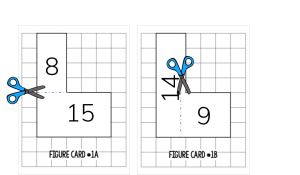Create figures and label each as a “yard”. Your students will work to find the perimeter around each yard so that a fence can be built around the property.

Consider telling your students that you are working on constructing fences around yards for “caterpillar families”. Why? Because the context of a yard with a fence is easy for students to visualize and understand but you will want to use much smaller numbers than you would find around a traditional yard so that your students can focus on the concept of area rather than the calculation of adding multiple large numbers together!

For each home, tell your students that you want to build a fence around the perimeter of the space and that to find the perimeter you will need to find the measurement of the outside of the entire figure by adding all of the sides together. Ask your students how they might accomplish this and work together to find the calculation.

Questions you might ask along the way would include:

• How might we calculate the perimeter of this figure?
• Have we added all of the sides together? How do we know we didn’t miss any?
• What might be the easiest way to add all of these numbers together?
• Are there any sides that are easier to add together first?
• What is the perimeter of the entire figure?
• What would the length of the fence around this property be?
• Can we use 1” toothpicks to prove our thinking?

Use a variety of yards including rectangles where all sides are labeled, a yard that is laid out on a grid, other shapes like triangles and rectilinear figures as well.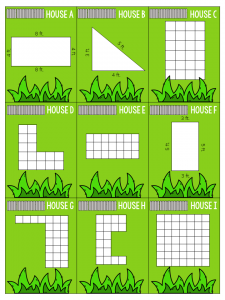Begin the lesson by telling your students that your caterpillar friends from lesson #3 now want to put a pool in their yard. Why caterpillars? The numbers we are using in these lessons are small so that your students are focused on concept rather than calculation, however, the measurements don’t make sense for a human sized yard and pool! They are trying to decide which pool might be best.

Your caterpillar friends know that they want a pool with an area of 10 sq. ft. but they aren’t sure about the dimensions of the length and width of their pool. Your job is to use square tiles to find all of the different rectangular configurations of 10 sq. ft. that they could create.

Allow your students to experiment with the square tiles. On chart paper, record the dimension, area and perimeter of the different pools your students were able to create. In this case you will have a 1 x 10 (or 10 x 1) pool with an area of 10 sq. ft and a perimeter of 22 ft. and also a 2 x 5 (or 5 x 1) pool with an area of 10 sq. ft. and a perimeter of 14 ft.

Ask your students “Are the areas of the pools the same? Are the perimeters of the pools the same? What do you notice?

Provide each of your students (or even set of partners) with their own area measurement and a set of square tiles. Allow your students to continue to explore on their own to find all possible rectangles that can be made from a specific area measurement and to make observations about the perimeter in each of these rectangles.

# Tools To USe